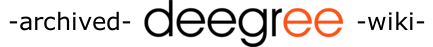[FrontPage] [TitleIndex] [WordIndex]

# Text2Tiff

## 1. General Information

First of all, please make sure to read the chapter on General Information for all deegreeTools.

## 2. Description

This class converts geodata and special values from a simple text file format to a .tiff image. The values are written as 32 bit float values.

## 3. Library Dependancies

• ```deegree2.jar
jai_codec.jar
jai_core.jar
mlibwrapper_jai.jar
batik-transcoder.jar
batik-util.jar
batik-gvt.jar
batik-extension.jar
batik-ext.jar
batik-awt-util.jar```

## 4. Usage

The program will be invoked as follows:

```java -Xms500m -Xmx1200m -classpath .;libs/deegree2.jar org.deegree.tools.raster.Text2Tiff
-r 1 -h -c 3  D:\deegree\data\myInput.xyz D:\deegree\data\myOutput```

command line parameters shall be used as follows:

--help, -help

• print this message

• Do/Do not read a header line in the input file. If enabled, one can specify column names instead of column numbers as seen below. Default is no.

--column-number n, -c n

• Use the column number n as input column. Must be specified if column name below is not given. Counting starts with one, so '3' means actually the third column, not the fourth.

--column-name n, -cn n

• Use the column named n as input column. Must be specified if no column number is given.

--oracle, -o

• Write the worldfile as Oracle expects it, using the outer bounds of the bbox and not the point centers. Default is no.

--image-type n

• n can be either 16 or 32. If n is 16, an image of type USHORT will be created, and the values will be stored as shorts, multiplied by 10. If n is 32, the float values will be stored in an image of type integer, as can be seen in Java's Float.floatToIntBits() method. Default is 16.

--image-width n | --image-height n

• If set, an image of this size will be created. If not set (default), the size will be determined by the resolution either determined automatically or set by hand.

--offset n

• use this as offset value for the result tiff. If result tiff shall be a 16Bit tiff first offset will be added to value before it will be multiplied by 10

--resolution n, -r n

• Set geo resolution to n. If omitted, the resolution will be set to the smallest value found in the input data.

--interpolate, i

• Interpolate missing values. By default, no interpolation will be performed.

--interpolate-power n

• Interpolate using n as power. Default is " + interpolatePower + ".

--interpolate-min-data n

• Interpolate only in the presence of n values within the search radius. Default is " + interpolateMinData + ".

--interpolate-max-data n

• Interpolate using a maximum of n values from within the search radius. If more values are found, the n nearest will be used. Default is " + interpolateMaxData + ".

--interpolate-no-value n

• The value to be used if insufficient data is in the search radius. See also the radius-increase options below. Default is " + interpolateNoValue + ".

• Interpolate using a search radius of n in the x direction. Default is " + interpolateRadiusX + ".

• Interpolate using a search radius of n in the y direction. Default is " + interpolateRadiusY + ".

• Automatically increase the x search radius by n if less than --i-min-data values are found. If specified and not 0, the value --i-no-value will be ignored. Default is 0.

• Automatically increase the y search radius by n if less than --i-min-data values are found. If specified and not 0, the value --i-no-value will be ignored. Default is 0.

--interpolate-ignore-range min max

• Adds a new range of values to be ignored while interpolating.

<outputfile>

• The outputfile parameter will be extended with ".tif" or ".tfw".

You may also be interested the SimpleText2Tiff tool.

look for other deegreeTools

2018-04-20 12:04